## Reflection and Mirrors Review

### Part A: Multiple Choice

1. As the angle of incidence is increased for a ray incident on a reflecting surface, the angle between the incident and reflected rays ultimately approaches what value?

 a. zero b. 45 degrees c. 90 degrees d. 180 degrees

2. If you stand three feet in front of a plane mirror, how far away would you see yourself in the mirror?

 a. 1.5 ft b. 3.0 ft c. 6.0 ft d. 12.0 ft

3. A concave mirror with a focal length of 10.0 cm creates a real image 30.0 cm away on its principal axis; the corresponding object is located how far from the mirror?

 a. 20.0 cm b. 15.0 cm c. 7.5 cm d. 5.0 cm

4. A concave mirror forms a real image at 25.0 cm from the mirror surface along the principal axis. If the corresponding object is at a 10.0 cm distance, what is the mirror's focal length?

 a. 1.4 cm b. 16.7 cm c. 12.4 cm d. 7.1 cm

5. If a virtual image is formed along the principal axis 10.0 cm from a concave mirror with the focal length 15.0 cm, what is the object distance from the mirror?

 a. 30.0 cm b. 10.0 cm c. 12.4 cm d. 6.0 cm

6. If a virtual image is formed 10.0 cm along the principal axis from a convex mirror of focal length -15.0 cm, what is the object distance from the mirror?

 a. 30.0 cm b. 10.0 cm c. 6.0 cm d. 3.0 cm

7. If a man's face is 30.0 cm in front of a concave shaving mirror creating an upright image 1.50 times as large as the object, what is the mirror's focal length?

 a. 12.0 cm b. 20.0 cm c. 70.0 cm d. 90.0 cm

8. Which of the following best describes the image formed by a plane mirror?

1. virtual, inverted and enlarged
2. real, inverted and reduced
3. virtual, upright and the same size as object
4. real, upright and the same size as object

9. Which of the following best describes the image formed by a concave mirror when the object is located somewhere between the focal point (F) and the center of curvature (C) of the mirror?

 a. virtual, upright and enlarged b. real, inverted and reduced c. virtual, upright and reduced d. real, inverted and enlarged

10. Which of the following best describes the image formed by a concave mirror when the object is at a distance further than the center of curvature (C) of the mirror?

 a. virtual, erect and enlarged b. real, inverted and reduced c. virtual, upright and reduced d. real, inverted and enlarged

11. Which of the following best describes the image formed by a concave mirror when the object distance from the mirror is less than the focal length (f)?

 a. virtual, upright and enlarged b. real, inverted and reduced c. virtual, upright and reduced

12. Which of the following best describes the image formed by a convex mirror when the object distance from the mirror is less than the absolute value of the focal length (f)?

 a. virtual, upright and enlarged b. real, inverted and reduced c. virtual, upright and reduced d. real, inverted and enlarged

13. Use of a parabolic mirror, instead of one made of a circular arc surface, can be used to reduce the occurrence of which of the following effects?

 a. spherical aberration b. mirages c. chromatic aberration d. light scattering

14. When the image of an object is seen in a plane mirror, the image is ____.

 a. real and upright. b. real and inverted. c. virtual and upright. d. virtual and inverted.

15. When the image of an object is seen in a plane mirror, the distance from the mirror to the image depends on ____.

1. the wavelength of light used for viewing.
2. the distance from the object to the mirror.
3. the distance of both the observer and the object to the mirror.

16. If a man wishes to use a plane mirror on a wall to view both his head and his feet as he stands in front of the mirror, the required length of the mirror ____.

1. is equal to the height of the man.
2. is equal to one half the height of the man.
3. depends on the distance the man stands from the mirror.
4. depends on both the height of the man and the distance from the man to the mirror.

17. When the image of an object is seen in a concave mirror the image will ____.

 a. always be real. b. always be virtual. c. be either real or virtual. d. will always be magnified.

18. When the image of an object is seen in a convex mirror the image will ____.

 a. always be real. b. always be virtual. c. may be either real or virtual. d. will always be magnified.

19. Rays of light traveling parallel to the principal axis of a concave mirror will come together ____.

 a. at the center of curvature. b. at the focal point. c. at infinity. d. at a point half way to the focal point.

20. Right angle mirrors produce three images of objects. Which of the following is statements is/are true of the middle image?

1. The middle image is the same size as the object.
2. The middle image is the same distance from the mirror as the object.
3. The middle image will exhibit left-right reversal relative to the object.
4. The middle image is a real image.
5. The middle image is an upright image.
6. The magnification of the middle image is -1.
7. When sighting at the middle image, light will reflect twice prior to reaching one's eye.

21. Two plane mirrors are attached to form a dual mirror system with an adjustable angle. As the angle between the mirrors increases, the number of images _____.

 a. increase b. decreases c. remains the same

22. Which of the following statements is/are true of a virtual image?

1. Virtual images are always located behind the mirror.
2. Virtual images can be either upright or inverted.
3. Virtual images can be magnified in size, reduced in size or the same size as the object.
4. Virtual images can be formed by concave, convex and plane mirrors.
5. Virtual images are not real; thus you could never see them when sighting in a mirror.
6. Virtual images result when the reflected light rays diverge.
7. Virtual images can be projected onto a sheet of paper.

23. Which of the following statements is/are true of a real image?

1. Real images are always located behind the mirror.
2. Real images can be either upright or inverted.
3. Real images can be magnified in size, reduced in size or the same size as the object.
4. Real images can be formed by concave, convex and plane mirrors.
5. Real images are not virtual; thus you could never see them when sighting in a mirror.
6. Real images result when the reflected light rays diverge.
7. Real images can be projected onto a sheet of paper.

24. Which of the following situations would always result in an image which is magnified in size?

1. Concave mirror: di > 0 and do < di
2. Convex mirror: do > 0
3. Plane Mirror: do > 0
4. Concave Mirror: do> R
5. Concave Mirror: do< f
6. Concave Mirror: do> f and do < R

25. Which of the following situations would always result in an image which is upright?

1. Concave mirror: di > 0 and do < di
2. Convex mirror: do > 0
3. Plane Mirror: do > 0
4. Concave Mirror: do> R
5. Concave Mirror: do< f
6. Concave Mirror: do> f and do < R

26. TRUE or FALSE:

Virtual images formed by mirrors are always upright images.
 a. TRUE b. FALSE

### Part B: Diagramming

27. On the diagram below, sketch the path the light ray would take as it travels.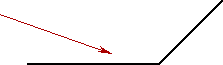28. Locate the images of the objects below and state which images can be seen by the eye.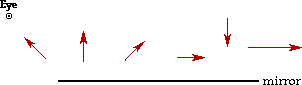29. Locate all of the images formed by the mirror systems below.30. Consider the following object in front of a plane mirror. Construct a ray diagram to show how light travels from the object (arrow) to the mirror and ultimately to the eye; then indicate the portion of the mirror needed in order for the eye to view the image.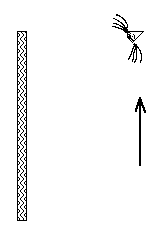31. For the right angle mirror below, draw in the secondary (or middle) image and show how light travels from the middle image to the eye.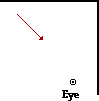32. Construct ray diagrams to show where the images of the following objects are located. Dray in the complete image and describe its characteristics (real or virtual, enlarged or reduced in size, inverted or upright). (NOTE: review the ray diagrams for all possible objects locations for each device.)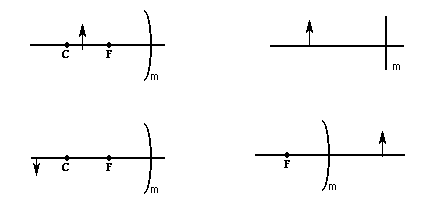### Part C: Calculations and Explanations

33. Distinguish between diffuse and regular (specular) reflection in terms of both cause and result.

34. Write the formulae which show the relationship between image distance, object distance and focal length, and the magnification of an object/image.

35. Fill in the blanks below for the problem-solving rules:

Focal lengths, object and image distances are ________ if they are on the reflective side of the mirror. Object and image heights are positive if they are ________ the principle axis.

36. What is the focal length of a mirror which gives an image 3.00 meters away when an object is placed 150. cm from it?

37. Where is the image located when an object is placed 60.0 cm from a mirror which has a focal length of 20.0 cm?

38. How far would an object need to be placed from a mirror of focal length 10 cm if it is to produce an image which is 20.0 cm BEHIND the mirror?

39. A mirror with a focal length of -100.0 cm is used to form an image. An object is placed 50.0 cm in front of the mirror.

a. Where is the image located?

b. What type of mirror is being used in this problem?

40. An object is placed 20.0 cm from a mirror of focal length 10.0 cm. The object is 5.00 cm tall. Where is the image located? How tall is the image?

41. A 20.0 cm tall object produces a 40.0 cm tall real image when placed in front of a curved mirror with a focal length of 50.0 cm. Determine the place that the object must be located to produce this image.

42. A mirror is used to produce a virtual image that is 5.00 times bigger than the object. If the focal length of the mirror is 100. cm, where will the image be located?

#### Navigate to:

Review Session Home - Topic Listing

Reflection and Mirrors - Home || Printable Version || Questions with Links

Answers to Questions:  All || #1-#19 || #20-#26 || #27-#32 || #33-#42

## You Might Also Like ...

Users of The Review Session are often looking for learning resources that provide them with practice and review opportunities that include built-in feedback and instruction. If that is what you're looking for, then you might also like the following:

The Calculator Pad includes physics word problems organized by topic. Each problem is accompanied by a pop-up answer and an audio file that explains the details of how to approach and solve the problem. It's a perfect resource for those wishing to improve their problem-solving skills.

Visit: The Calculator Pad Home | Calculator Pad - Reflection and Mirrors

2. Minds On Physics the App Series

Minds On Physics the App ("MOP the App") is a series of interactive questioning modules for the student that is serious about improving their conceptual understanding of physics. Each module of the series covers a different topic and is further broken down into sub-topics. A "MOP experience" will provide a learner with challenging questions, feedback, and question-specific help in the context of a game-like environment. It is available for phones, tablets, Chromebooks, and Macintosh computers. It's a perfect resource for those wishing to refine their conceptual reasoning abilities. Part 6 of the series includes topics in Reflection and Mirrors.

Visit: MOP the App Home || MOP the App - Part 6